# IB DP Physics: SL复习笔记2.4.2 Impulse

### Impulse

• The force and momentum equation can be rearranged to find the impulse
• Impulse, I, is equal to the change in momentum:

I = FΔt = Δp = mv – mu

• Where:
• I = impulse (N s)
• F = force (N)
• t = time (s)
• p = momentum (kg m s–1)
• m = mass (kg)
• v = final velocity (m s–1)
• u = initial velocity (m s–1)
• This equation is only used when the force is constant
• Since the impulse is proportional to the force, it is also a vector
• The impulse is in the same direction as the force
• The unit of impulse is N s
• The impulse quantifies the effect of a force acting over a time interval
• This means a small force acting over a long time has the same effect as a large force acting over a short time

#### Rain vs Hail

• An example in everyday life of impulse is the effect of rain on an umbrella, compared to hail (frozen water droplets)
• When rain hits an umbrella, the water droplets tend to splatter and fall off it and there is only a very small change in momentum
• However, hailstones have a larger mass and tend to bounce back off the umbrella, creating a greater change in momentum
• Therefore, the impulse on an umbrella is greater in hail than in rain
• This means that more force is required to hold an umbrella upright in hail compared to rain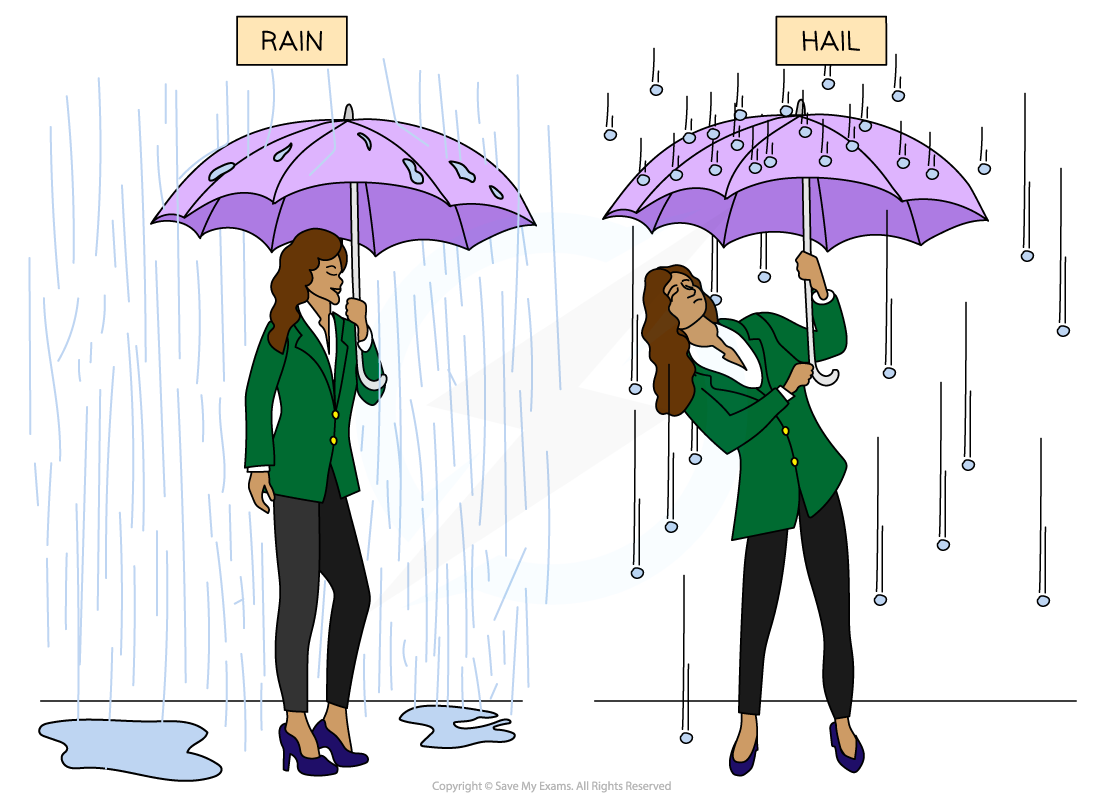Since hailstones bounce back off an umbrella, compared to water droplets from rain, there is a greater impulse on an umbrella in hail than in rain

#### Worked Example

A 58 g tennis ball moving horizontally to the left at a speed of 30 m s–1 is struck by a tennis racket which returns the ball back to the right at 20 m s–1.(a) Calculate the impulse delivered to the ball by the racket(b) State the direction of the impulse

Part (a)

Step 1: Write the known quantities

• Taking the initial direction of the ball as positive (the left)
• Initial velocity, u = 30 m s–1
• Final velocity, v = –20 m s–1
• Mass, m = 58 g = 58 × 10–3 kg

Step 2: Write down the impulse equation

Impulse I = Δp = m(v – u)

Step 3: Substitute in the values

I = (58 × 10–3) × (–20 – 30) = –2.9 N s

Part (b)

Direction of the impulse

• Since the impulse is negative, it must be in the opposite direction to which the tennis ball was initial travelling (since the left is taken as positive)
• Therefore, the direction of the impulse is to the right

#### Impulse on a Force-Time Graph

• In real life, forces are often not constant and will vary over time
• If the force is plotted against time, the impulse is equal to the area under the force-time graph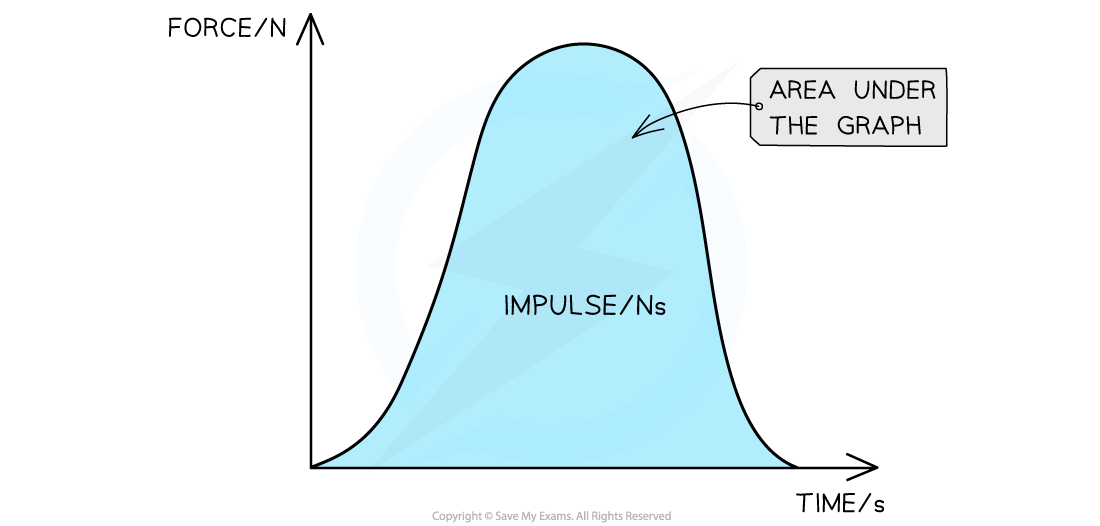When the force is not constant, the impulse is the area under a force–time graph

• This is because

Impulse = FΔt

• Where:
• F = force (N)
• Δt = change in time (s)
• The impulse is therefore equal whether there is
• A small force over a long period of time
• A large force over a small period of time
• The force-time graph may be a curve or a straight line
• If the graph is a curve, the area can be found by counting the squares underneath
• If the graph is made up of straight lines, split the graph into sections
• The total area is the sum of the areas of each section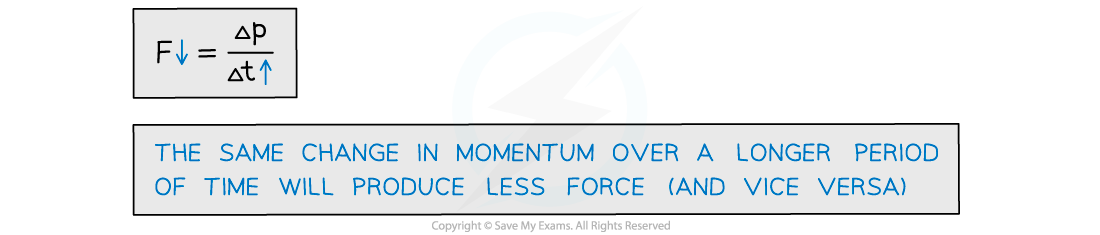#### Worked Example

A ball of mass 3.0 kg, initially at rest, is acted on by a force F which varies with t as shown by the graph.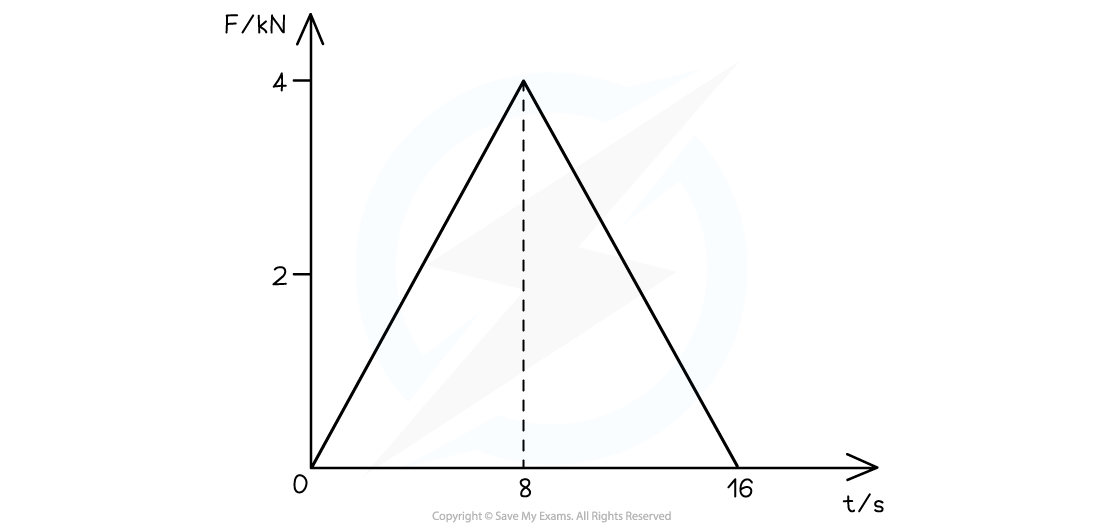Calculate the magnitude of the velocity of the ball after 16 s.

Step 1: List the known quantities

• Mass, m = 3.0 kg
• Initial velocity, u = 0 m s−1 (since it is initially at rest)

Step 2: Calculate the impulse

• The impulse is the area under the graph
• The graph can be split up into two right-angled triangles with a base of 8 s and a height of 4 kNArea = Impulse = 32 × 103 N s

Step 3: Write the equation for impulse

Impulse, I = Δp = m(v – u)

Step 4: Substitute in the values

I = mv

32 × 103 = 3.0 × v

v = (32 × 103) ÷ 3.0

v = 10 666 m s–1 = 11 km s–1

Step 5: State the final answer

• The final magnitude of the velocity of the ball is:

v = 11 km s–1

#### Exam Tip

Remember that if an object changes direction, then this must be reflected by the change in sign of the velocity. As long as the magnitude is correct, the final sign for the impulse doesn't matter as long as it is consistent with which way you have considered positive (and negative)For example, if the left is taken as positive and therefore the right as negative, an impulse of 20 N s to the right is equal to −20 N sSome maths tips for this section:Rate of Change

• ‘Rate of change’ describes how one variable changes with respect to another
• In maths, how fast something changes with time is represented as dividing by Δt (e.g. acceleration is the rate of change in velocity)
• More specifically, Δt is used for finite and quantifiable changes such as the difference in time between two events

Areas

• The area under a graph may be split up into different shapes, so make sure you’re comfortable with calculating the area of squares, rectangles, right-angled triangles and trapeziums!

### Impulse in Context

#### In Sports

• For example, in cricket:
• A cricket ball travels at very high speeds and therefore has a high momentum
• When a fielder catches the ball, it exerts a force onto their hands
• Stopping a ball with high momentum at once will cause a large force onto their hands
• This is because a change in momentum (impulse) acts over a short period of time which creates a large force on the fielder's hands and could cause serious injury
• A fielder moves their hands back when they catch the ball, which increases the time for its change in momentum to reduce
• This means there will be less force exerted on the fielder's hands and therefore less chance of injury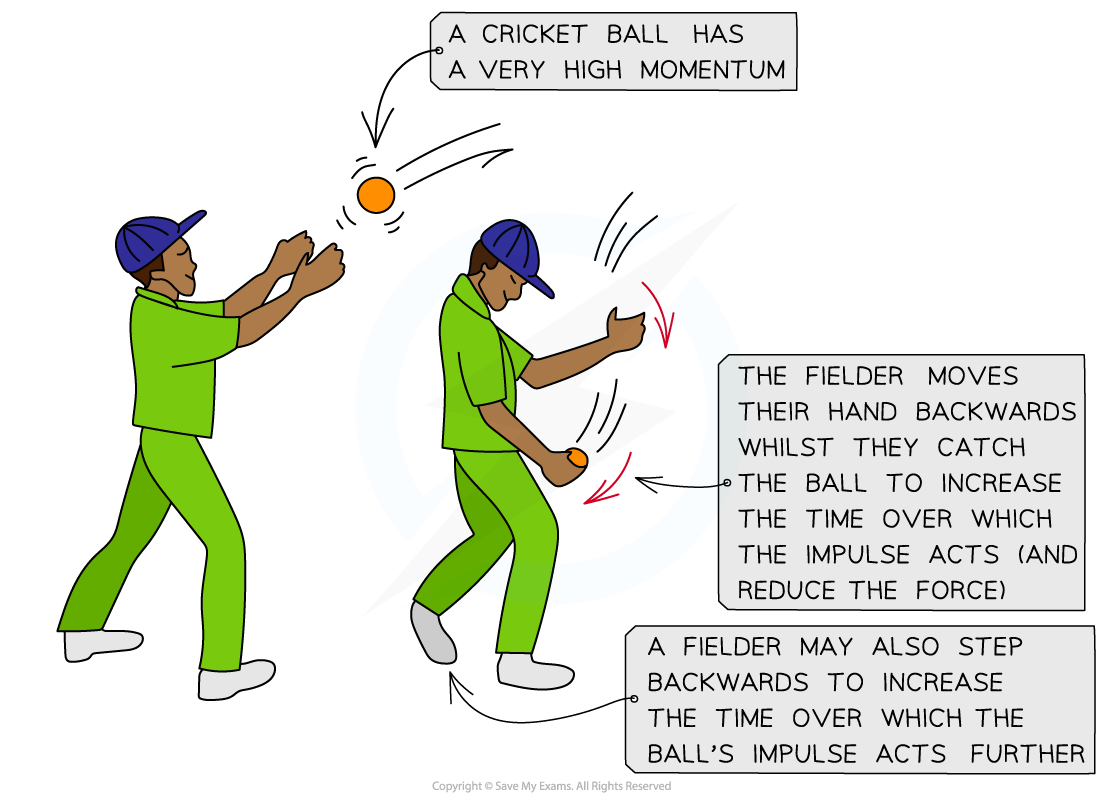A cricket fielder moves their hands backwards when catching a cricket ball to reduce the force it will exert on their hands

• In football:
• Increasing the contact time is sometimes used to advantage, as the longer the contact time, the larger change in momentum
• When kicking a football, after a strong kick the motion is followed through
• This creates a large impulse and the ball then has a higher velocity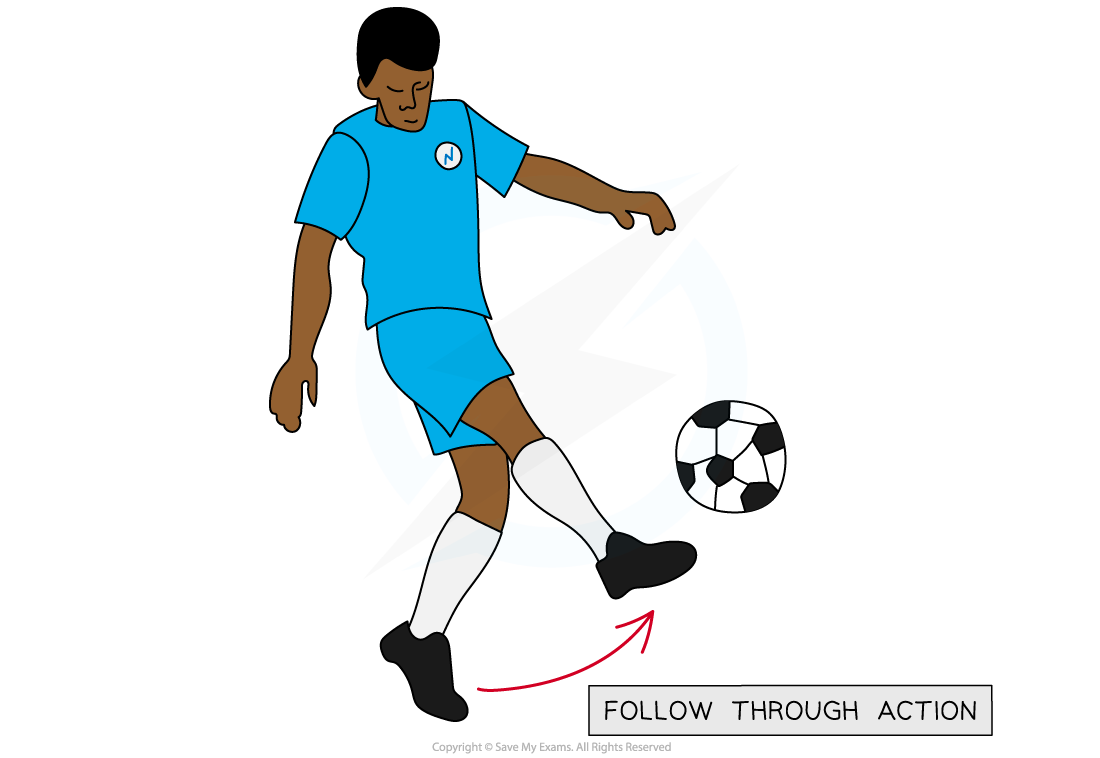The follow through action of a football kick increases the change in momentum of the ball

#### Worked Example

A tennis ball hits two rackets with a change in momentum of 0.5 kg m s−1. The first racket has a contact time of 2 s. The second racket has a contact time of 0.1 s.For the different contact times, which tennis racket experiences more force from the tennis ball?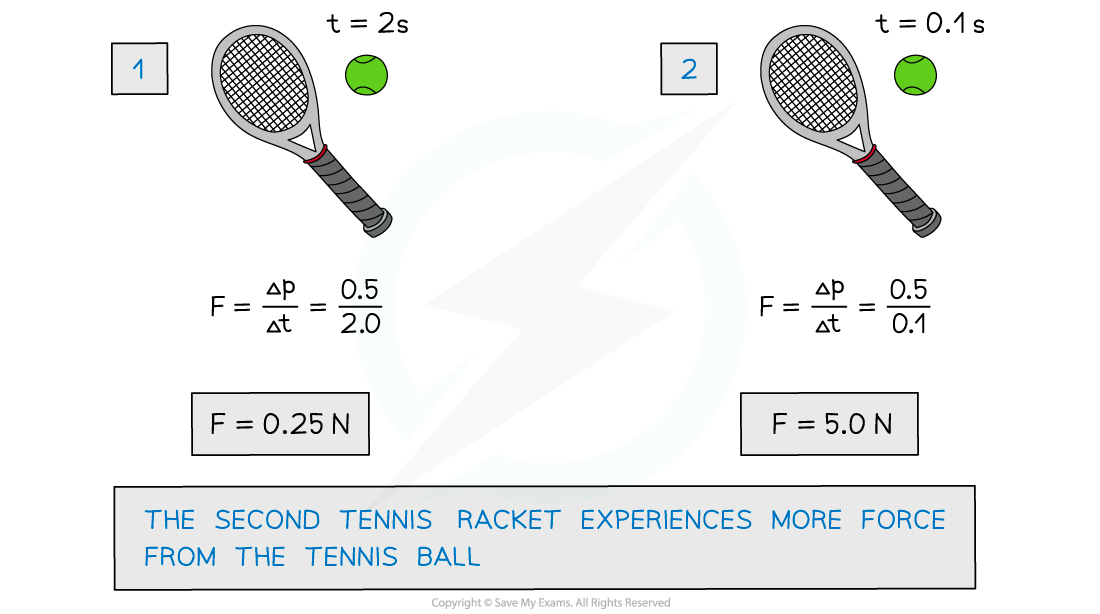#### Momentum Conservation & Safety

• The force of an impact in a vehicle collision can be decreased by increasing the contact time over which the collision occurs
• The contact time is the time in which the vehicle or the passenger is in contact with what it has collided with
• Vehicles have safety features such as crumple zones, seat belts and airbags to account for this
• For a given force upon impact, these absorb the energy from the impact and increase the time over which the force takes place
• This, in turn, increases the time taken for the change in momentum of the passenger and the vehicle to come to rest
• The increased time reduces the force and risk of injury on a passenger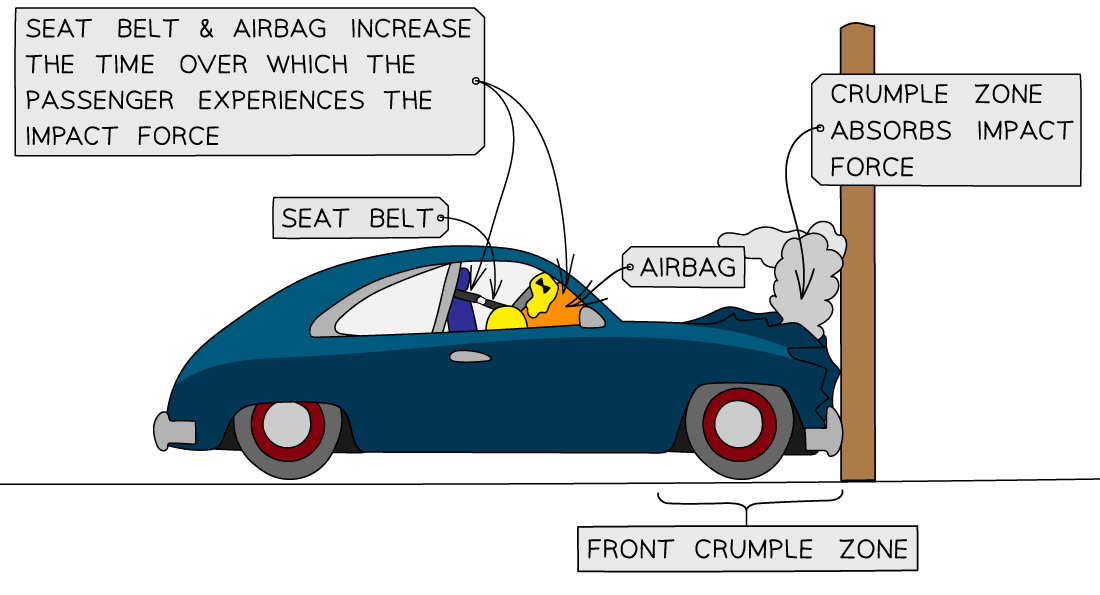The seat belt, airbag and crumple zones help reduce the risk of injury on a passenger

#### Designing Safety Features

• Vehicle safety features are designed to absorb energy upon an impact by changing shape
• Seat belts
• These are designed to stop a passenger from colliding with the interior of a vehicle by keeping them fixed to their seat in an abrupt stop
• They are designed to stretch slightly to increase the time for the passenger's momentum to reach zero and reduce the force on them in a collision
• Airbags
• These are deployed at the front on the dashboard and steering wheel when a collision occurs
• They act as a soft cushion to prevent injury on the passenger when they are thrown forward upon impact
• Crumple zones
• These are designed into the exterior of vehicles
• They are at the front and back and are designed to crush or crumple in a controlled way in a collision
• This is why vehicles after a collision look more heavily damaged than expected, even for relatively small collisions
• The crumple zones increase the time over which the vehicle comes to rest, lowering the impact force on the passengers
• The effect of the increase in time and force can be shown on a force-time graph
• For the same change in momentum, which depends on the mass and speed of a vehicle, the increase in contact time will result in a decrease in the maximum force exerted on the vehicle and passenger
• This is demonstrated by a lower peak and wider base on a force-time graph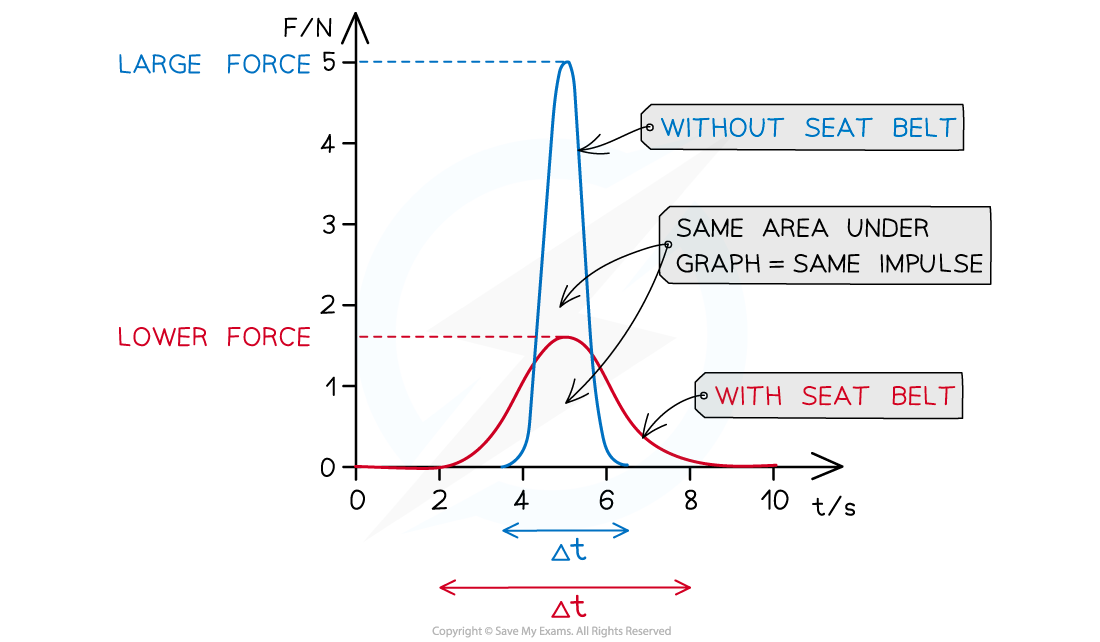The increase in contact time Δt decreases the force for the same impulse

#### Worked Example

A 7 kg bowling ball has an impulse of 84 N s act upon it. The bowling ball was initially at rest and sitting on a flat frictionless surface.Predict the distance moved by the bowling ball in the first 3 seconds after the impulse was delivered.

Step 1: List the known quantities

• Mass of the bowling ball, m = 7 kg
• Impulse acting on the bowling ball, I = 84 N s
• Bowling ball initial velocity (at rest), u = 0 m s−1
• Time of movement, t = 3 s

Step 2: Find the velocity caused by the impulse

• The velocity caused by the impulse can be found from the equation linking mass, velocity, and impulse:

Impulse, I = Δp = m(v – u)

Step 3: Rearrange and solve for v

I = m × v (since u = 0 m s−1)

v = I ÷ m = 84 ÷ 7 = 12 m s−1

Step 4: Find the distance travelled

• This can be found using time and velocity

v = d ÷ t

d = v × t

d = 12 × 3 = 36 m

Step 5: State the final answer

• The bowling ball moved 36 m